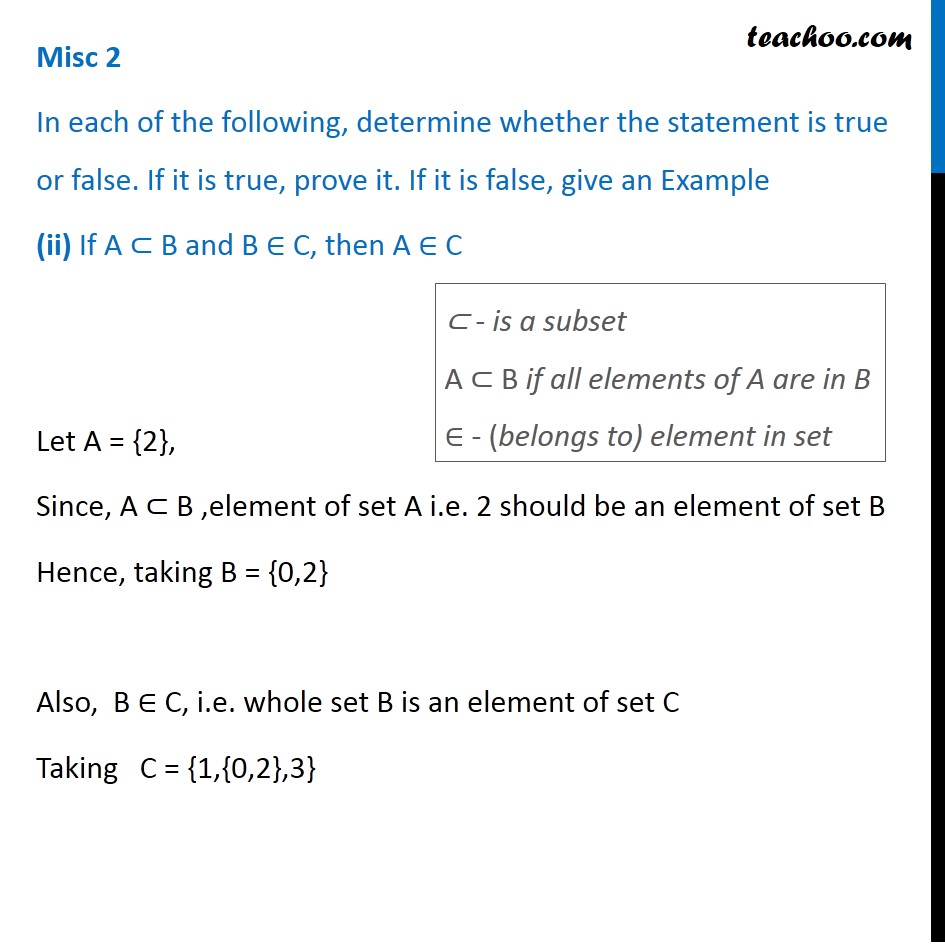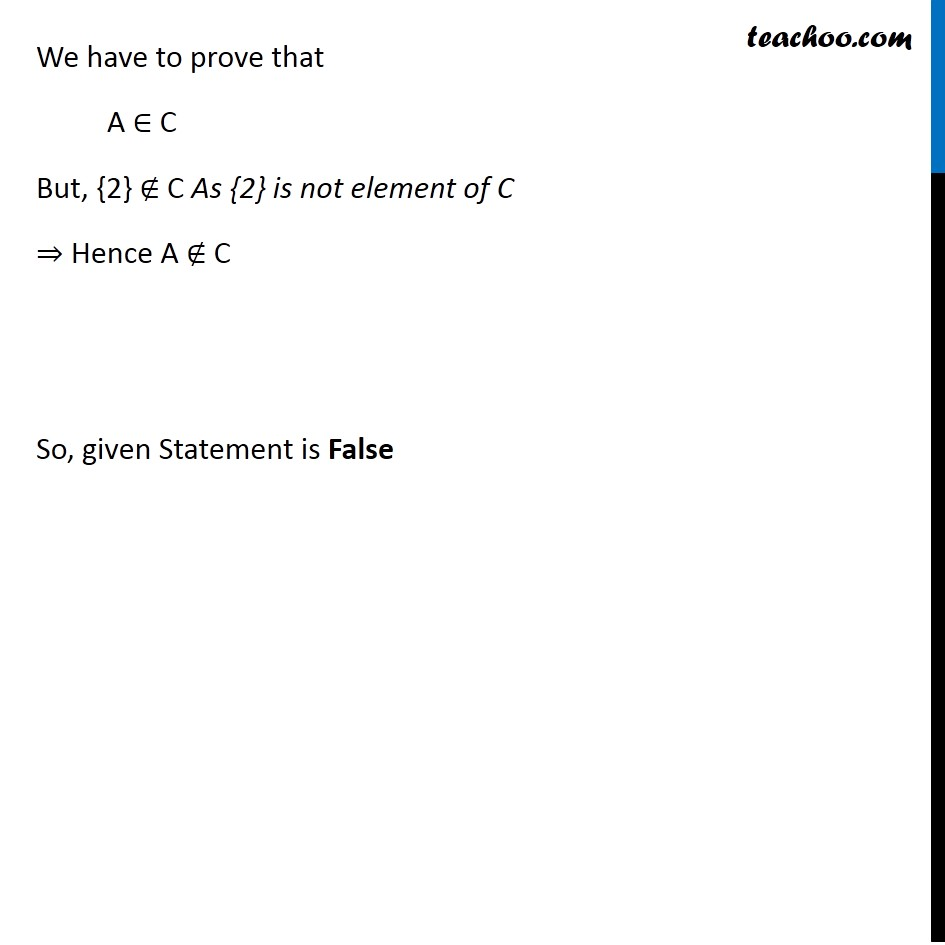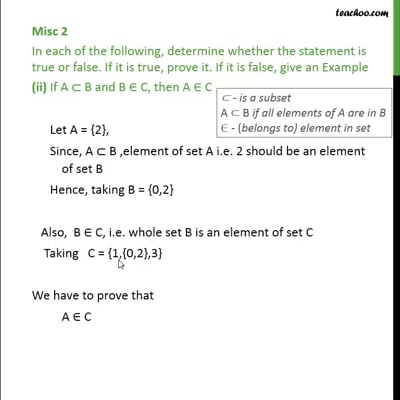Miscellaneous

Chapter 1 Class 11 Sets
Serial order wiseThis video is only available for Teachoo black users

Introducing your new favourite teacher - Teachoo Black, at only ₹83 per month

### Transcript

Misc 2 In each of the following, determine whether the statement is true or false. If it is true, prove it. If it is false, give an Example (ii) If A ⊂ B and B ∈ C, then A ∈ C Let A = {2}, Since, A ⊂ B ,element of set A i.e. 2 should be an element of set B Hence, taking B = {0,2} Also, B ∈ C, i.e. whole set B is an element of set C Taking C = {1,{0,2},3} ⊂ - is a subset A ⊂ B if all elements of A are in B ∈ - (belongs to) element in set We have to prove that A ∈ C But, {2} ∉ C As {2} is not element of C ⇒ Hence A ∉ C So, given Statement is False